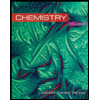# HEAT OF NEUTRALIZATION NAME: POST LABORATORY QUESTIONS 1. Specific to this experiment, list two reasons why your data contains an experimental error. a. b. 2. A 15.00 gram sample of ammonium chloride (NH4CI) was dissolved in 100.00 grams of water. How much heat is produced by the dissolution (absorbed from the solution) if the solution temperature goes from 22.0 oC to 1.0 oC. The specific heat of the solution is 4.18 J/g °C 3. 300.0 mL of a 2.500 M solution X was mixed with 300.0 mL of a 2.500 M solution Y in a calorimeter. Both of the solutions were at the same temperature initially. Determine the heat of neutralization (kJ/mole) if the temperature goes from 12.0 °C to 29.0 °C. Assume a molar ratio of 1:1.The specific heat of the solution is 4.281 J/g °C. The density of the mixture is 1.050 g/mL. 4. Sketch a crude time-temperature graph for an endothermic reaction.

Question

Question 3

Expert Solution

### Want to see the full answer?

Check out a sample Q&A hereStudents who’ve seen this question also like:Chemistry
10th Edition
ISBN: 9781305957404
Author: Steven S. Zumdahl, Susan A. Zumdahl, Donald J. DeCoste
Publisher: Cengage Learning